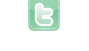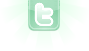## 5 Squares and per Square -10 size

I need help i became a task from my teacher and I dont know how to do it, can u help me ? Here is the task: Now create five Squares objects with a while loop, the size of which starts at 50 and decreases by 10 and which are placed from bottom left to top right. My current code is this: private void Prepare() { int h = 1; int x = 300; int y = 400; addObject(new Squares(false), 100,100); addObject(new Squares(true), 700,500); while (h < 6) { addObject(new Squares(true), x, y); x = x + 50; y = y - 50; h++; }
Well, that is a bit different from what we did in your previous discussion. You now need an constructor with an int size parameter:
```public Square()
{
this(50); // reroutes to another constructor
}

public Square(boolean randomSized)
{
this(randomSized ? 30+Greenfoot.getRandomNumber(50+1) : 50);
}

public Square(int size)
{
GreenfootImage img = new GreenfootImage(size, size);
img.setColor(getColor());
img.drawRect(0, 0, size-1, size-1);
setImage(img);
}```
Now, you can use new Square() or new Square(false) for a 50x50; you can use new Square(true) or new Square(30+Greenfoot.getRandomNumber(50+1)) for a random size; and you can use new Square(60-h*10) for your decreasing fixed sized squares.
Thanks a lot!